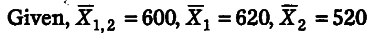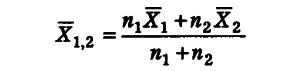# The mean monthly salary paid to all employees in a certain company was Rs. 600

The mean monthly salary paid to all employees in a certain company was Rs. 600. The mean monthly salaries paid to male and female employees were Rs. 620 and Rs. 520, respectively. Find the percentage of male to female employees in the company.Let n_{ 1 }= Number of male employees and n_{ 2 } = Number of female employees
Using the formula of combined arithmetic mean, we have600 = n_{ 1 }(620) + n_{ 2 }(520) /n_{ 1 }+n_{ 2 }
600(n_{ 1 }+n_{ 2 }) = 620\$n_{ 1 }\$ +520 n_{ 2 }
600\$n_{ 1 }\$+600\$n_{ 2 }\$ = 620\$n_{ 1 }\$ +520 n_{ 2 }
80\$n_{ 2 }\$ = 20 n_{ 1 }
n_{ 1 }/n_{ 2 } = 80/20=4/1
n_{ 1 } : n_{ 2 } = 4 : 1
Hence, the percentage of male employees =4/5 x 100 = 80 % and the percentage of female employees = 1/5 x 100=20%